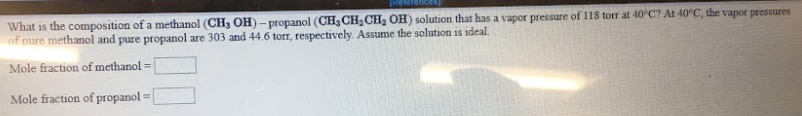# What is the composition of a methanol (CH3OH) - propanolol (CH3CH2CH2OH) solution that has a vapor pressure of 118 torr at 40 degrees C, the vapor pressures of pure methanol and pure propanolol are 303 and 44.6 torr, respectively. Assume the solution is ideal. Mole fraction of methanol = ___ Mole fraction of propanolol = ___